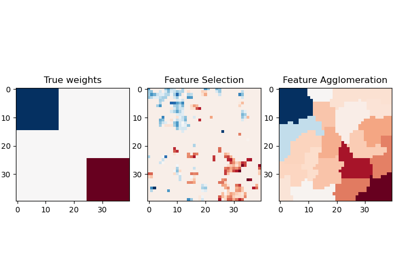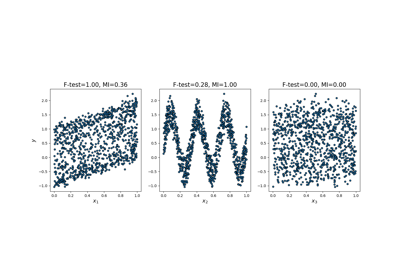# sklearn.feature_selection.f_regression¶

sklearn.feature_selection.f_regression(X, y, center=True)[source]

Univariate linear regression tests.

Linear model for testing the individual effect of each of many regressors. This is a scoring function to be used in a feature selection procedure, not a free standing feature selection procedure.

This is done in 2 steps:

1. The correlation between each regressor and the target is computed, that is, ((X[:, i] - mean(X[:, i])) * (y - mean_y)) / (std(X[:, i]) * std(y)).

2. It is converted to an F score then to a p-value.

For more on usage see the User Guide.

Parameters
X{array-like, sparse matrix} shape = (n_samples, n_features)

The set of regressors that will be tested sequentially.

yarray of shape(n_samples).

The data matrix

centerTrue, bool,

If true, X and y will be centered.

Returns
Farray, shape=(n_features,)

F values of features.

pvalarray, shape=(n_features,)

p-values of F-scores.

mutual_info_regression

Mutual information for a continuous target.

f_classif

ANOVA F-value between label/feature for classification tasks.

chi2

Chi-squared stats of non-negative features for classification tasks.

SelectKBest

Select features based on the k highest scores.

SelectFpr

Select features based on a false positive rate test.

SelectFdr

Select features based on an estimated false discovery rate.

SelectFwe

Select features based on family-wise error rate.

SelectPercentile

Select features based on percentile of the highest scores.

## Examples using sklearn.feature_selection.f_regression¶